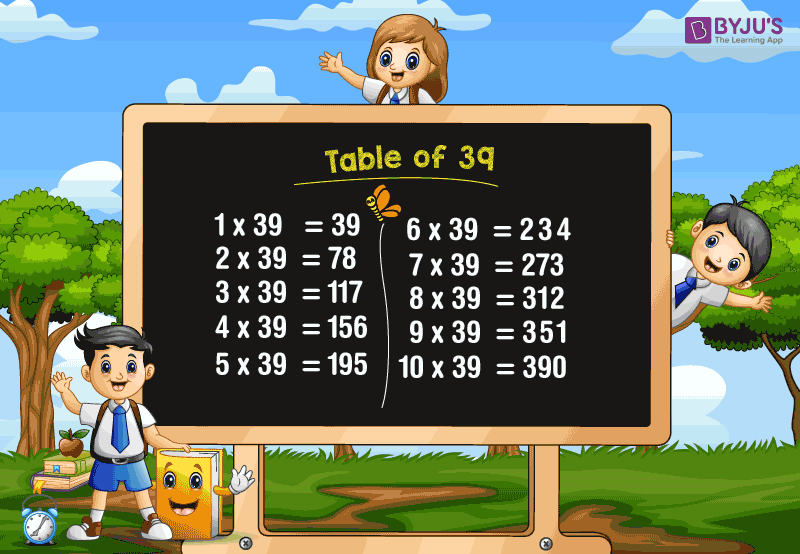# Table of 39

Learning the table of 39 is essential for students and helps in doing the higher-order calculations faster. Students are advised to memorize the table of 39, and it makes us perform well in the time-constrained examinations like CAT, GMAT, etc. In this article, we are going to learn the 39 times table using the chart and also learn how to read the table of 39.

### Download Table of 39 Here## 39 Times Table

The table of 39 is the multiplication table which shows the multiplication of the number 39 with whole numbers. The 39 times table gives the multiples of 39. Also, we will get a table of 39 if we repeatedly add the number for the number of times.

For example, 39 times 5 is 195.

It means that the multiplication of 39 and 5 is 195.

(i.e) 39 × 5 =195

Also, we will get the same result by adding the number 39 for 5 times.

(i.e.) 39+39+39+39+39 =195

Also, check tables from 1 to 100 for more details.

## Multiplication Table of 39

Go through the table of 39 given below and try to memorize it. The chart is provided with the multiplication of number 39 with different numbers (from 1 to 20) and solve the problems quickly.

 39 × 1 = 39 39 × 2 = 78 39 × 3 = 117 39 × 4 = 156 39 × 5 = 195 39 × 6 = 234 39 × 7 = 273 39 × 8 = 312 39 × 9 = 351 39 × 10 = 390 39 × 11 = 429 39 × 12 = 468 39 × 13 = 507 39 × 14 = 546 39 × 15 = 585 39 × 16 = 624 39 × 17 = 663 39 × 18 = 702 39 × 19 = 741 39 × 20 = 780

### How to Read 39 Times Table?

Read the table of 39 as follows:

• One time thirty-nine is 39
• Two times thirty-nine is 78
• Three times thirty-nine is 117
• Four times thirty-nine is 156
• Five times thirty-nine is 195
• Six times thirty-nine is 234
• Seven times thirty-nine is 273
• Eight times thirty-nine is 312
• Nine times thirty-nine is 351
• Ten times thirty-nine is 390
• Eleven times thirty-ninet is 429
• Twelve times thirty-nine is 468
• Thirteen times thirty-nine is 507
• Fourteen times thirty-nine is 546
• Fifteen times thirty-nine is 585.

Get More Maths Tables:

## Frequently Asked Questions on Table of 39

### 1. What is the table of 39?

The table of 39 is the multiplication of number 39 with the different whole numbers. The table of 39 is also called the 39 times tables, which gives the multiples of 39.

### 2. What is the value of 10 times 39?

10 times 39 is 390. (i.e.) 10 × 39 = 390.

### 3. What are the first 10 multiples of 39?

The first ten multiples of 39 are 39, 78, 117, 156, 195, 234, 273, 312, 351, and 390.

### 4. What is the cost of 7 apples, if each apple costs Rs. 39?

The cost of one apple is Rs. 39.
Therefore, the cost of 7 apples = 7 × 39 = 273.
Hence, the cost of 7 apples is Rs. 273.

### 5. Find the value of x, if 39x=585.

The value of x is 15.
Explanation: 39x= 585
x = 585/39
x =15.